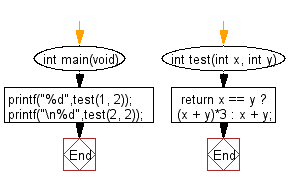﻿ C exercises: Compute the sum of the two given integer values. If the two values are the same, then return triple their sum - w3resource# C Exercises: Compute the sum of the two given integer values. If the two values are the same, then return triple their sum

## C-programming basic algorithm: Exercise-1 with Solution

Write a C program to compute the sum of the two given integer values. If the two values are the same, then return triple their sum.

C Code:

``````#include <stdio.h>
int main(void){
printf("%d",test(1, 2));
printf("\n%d",test(2, 2));
}
int test(int x, int y)
{
return x == y ? (x + y)*3 : x + y;
}
``````

Sample Output:

```3
12
```

Pictorial Presentation:Flowchart:C Programming Code Editor: